﻿ Employment Higher Degree B-Spline Function for Solving Higher Order Differential Equations

### Employment Higher Degree B-Spline Function for Solving Higher Order Differential Equations

Karwan H.F.Jwamer, Najim Abdullah I.

## Employment Higher Degree B-Spline Function for Solving Higher Order Differential Equations

Karwan H.F.Jwamer1,, Najim Abdullah I.2,1Mathematics Department, School of Science, University of Sulaimani, Sulaimani, Kurdistan Region, Iraq

2Mathematics Department, College of Science, University of Garmian, Kalar, Kurdistan Region, Iraq

### Abstract

As the B-spline method was developed for solving higher order differential equations, we present a brief survey to construct a higher degree B-spline. The new technique has been given in this field, accordingly a numerical illustration used to solve boundary value problems by employ quintic B-spline function. An example has been given for calculating maximum absolute error through n nodes.

• Karwan H.F.Jwamer, Najim Abdullah I.. Employment Higher Degree B-Spline Function for Solving Higher Order Differential Equations. International Journal of Partial Differential Equations and Applications. Vol. 4, No. 1, 2016, pp 16-19. http://pubs.sciepub.com/ijpdea/4/1/3
• H.F.Jwamer, Karwan, and Najim Abdullah I.. "Employment Higher Degree B-Spline Function for Solving Higher Order Differential Equations." International Journal of Partial Differential Equations and Applications 4.1 (2016): 16-19.
• H.F.Jwamer, K. , & I., N. A. (2016). Employment Higher Degree B-Spline Function for Solving Higher Order Differential Equations. International Journal of Partial Differential Equations and Applications, 4(1), 16-19.
• H.F.Jwamer, Karwan, and Najim Abdullah I.. "Employment Higher Degree B-Spline Function for Solving Higher Order Differential Equations." International Journal of Partial Differential Equations and Applications 4, no. 1 (2016): 16-19.

 Import into BibTeX Import into EndNote Import into RefMan Import into RefWorks

### 1. Introduction

B-spline is a spline function that has minimal support with respect to given degree, smoothness, and domain partition , and named B-splines because they formed a basis for all splines . Quartic spline solution of third order singularly perturbed B V P has been studied by . Theoretical background for stable computation by using B-splines with their derivatives studied by .  employ quartic B-spline collocation method for solving one-dimensional hyperbolic telegraph equation and exploitation. Quintic B-spline for the numerical solution of fourth order parabolic partial differential equations to find maximum error given by  while  discussed quartic B-spline differential quadrature method, and  employs quartic B-spline method to solve the self-adjoint boundary value problems.  in his paper approximate errors calculated by using cubic B-spline function.

As for us in this paper we construct a higher degree B-spline by two different method for solving self adjoint boundary value problems, in the following section we display deriving methods. Section 3 as example define a quintic B-spline. Section 4 describes the definition of Quintic B-spline. Finally Section 5 consists of a computer procedure to compute maximum error for several nodes.

### 2. Construction of B-Spline

If (ci)ni=1is a sequence of control points and x=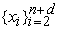is (n+d-1) knots for spline of degree d; we have seen that a typical spline can be written as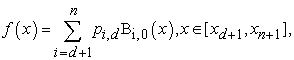where BI,0 is written as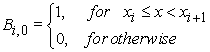(1)

Any spline of degree 0 can be expressed as a linear combination of the B-spline Bi,0.

And f(x)=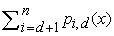is piecewise constant function and xd+1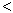xxn+1 although the end knots allowed to coincide.

So higher B-spline is generate fromlower degree of B-splines by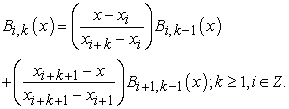(2)

The Bi,k functions as defined in (2) are called B-spline of degree k.

Another method to generate higher degree B-spline and it is valid only equidistant points:

The B-spline of order m is defined as follows: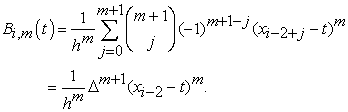Where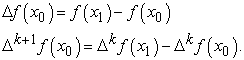### 3. Quintic B-spline

Let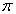be a uniform partition of the interval [0,1] such that a=x0<x1<…<xn=b where h=xi+1-xi or h=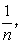then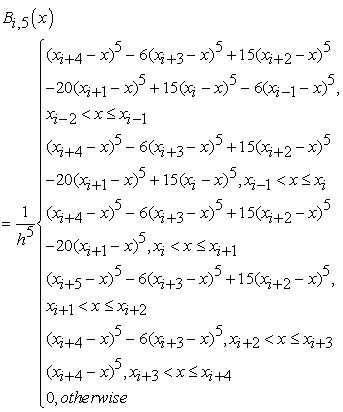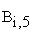(x) is the B-spline basis function of 5th degree which also called quintic B-spline vanish outside interval. Each quintic B-spline cover five elements. The basis function is non-zero on five knot spans. The set of quintic B-splines {B-3, B-2, B-1, … , BN, BN+1,BN+2} form a basis for the functions over interval [0, 1].

Now let s(x) be the B-spline interpolating function at the nodal points. Then s(x) can be written as s(x)=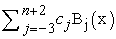where cj's are unknown coefficients and Bi(x)'s are quintic B-spline functions. The value of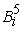at the nodal points can be obtained and its differentiating with respect to x, which are summarized in Table 2.

### 4. Description of the Method

Consider the self-adjoin fourth-order singularly perturbed boundary value problem of the form: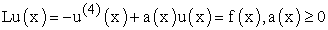(3)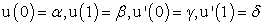(4)

Where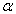,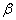,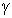and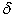are constants and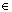is a small positive parameter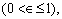a(x), and f(x) are sufficiently smooth functions. In this survey, we take a(x)=a= constant. Let u(x)= s(x)=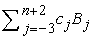be the approximate solution of boundary value problem (3). Then let x0,x1,…,xn be n+1 grid points in the interval [0,1]. So that we have, xi=x0+ih, x0=0, xn=1, i=1, 2, …, n; h=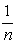at the knots, we get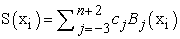(5)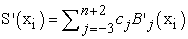(6)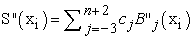(7)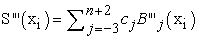(8)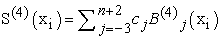(9)

Putting the value of equations (5)-(9) in equation (3), we get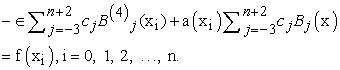(10)

And the boundary condition becomes,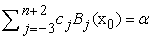(11)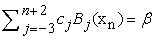(12)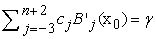(13)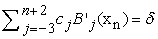(14)

The values of the spline function at the knots are determined using table (4.1) and substituting in equations (10)-(14) a system of (n+4) equations with (n+4) unknown. Now, we can write the above system of equations in the following form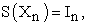where Xn=(c-3,c-2,c-1,…,c0,c1,…,cn+2)T are unknowns,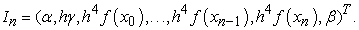From equation (10):

and boundary condition(11-14),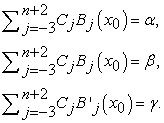, we get the following:

If i=0,then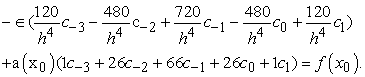(15)

For i=1, we obtain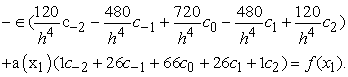(16)

For i=2, then we have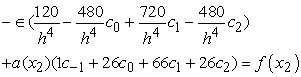(17)

For i=3, then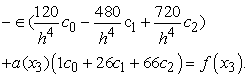(18)

If i=4, thus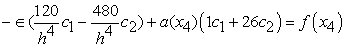(19)

If i=5, then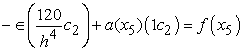(20)

For i=6 ,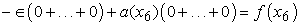(21)

For i-n-4, then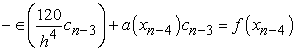(22)

For i=n-3, then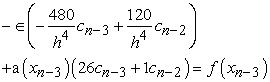(23)

For i-n-2, then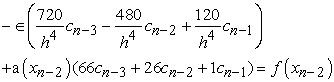(24)

For i=n-1, then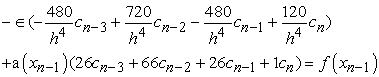(25)

Finally for i-n, we obtain that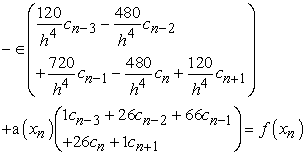(26)

And boundary conditions(11)-(14) gives: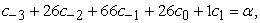(27)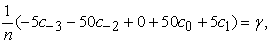(29)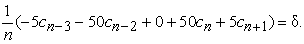(30)

### 5. Numerical Result

In this section we solve higher order B. V. Ps. By using quintic B-spline interpolation as follows:

For order four B. V. Ps. Take the following

Example 1: Consider the fourth order boundary value problem: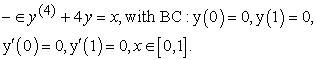The maximum error bound gives by the following table:

#### Table 1. Absolute maximum errors at given N=10, 20, 40 and ∈ =10-1, 10-2, 10-3

For order three B. V. Ps. Take the following

Example 2: Consider the following third order singular perturbation problem :The maximum error bound gives by the following table:

#### Table 2. Absolute maximum errors at given N=10, 20, 40 and ∈ =10-1, 10-2, 10-3

For order two B. V. Ps. Take the following

Example 3: Consider the second order boundary value problem with singular perturbation form: -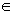y''+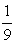y=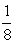x2 ,and subject to the boundary conditions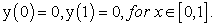The maximum error bound gives by the following table:

### 6. Conclusion

In this paper, we design higher order B –Spline to solve second, third, and fourth order singular perturbed boundary value problems. Also there examples are presented with different values of n and ∈ and they showed the efficiency and of our design .

### References

  AlperKorkmaz, A. Murat Aksoy andIdrisDağ, Quartic B-spline Differential Quadrature Method, International Journal of Nonlinear Science, Vol. 11, (2011) No.4,pp.403-411.In article  GhazalaAkram, Quantic Spline Solution of a third Singularly Perturbed b. v. p., ANZIMTJ, 53, 2012, 44-53.In article  JalilRashidinia and Sharifi, Survey of B-spline Function to Approximate the solution of mathematical problems.In article  MarziehDosti, ALirezaNazemi Quantic B-SplineCollocation Method for Solving One-dimensional Hyperbolic Telegraph Equation, Department of Mathematics school of Mathematical. Science, Sharhood University of Technology, sharhood, Iran, Journal of Information and Computing Science Vol.7 No2, 2012, PP. 083-090.In article  Shahid S. Siddiqi and SaimaArshed Quantic B-Spline for the Numerical Solution of 4th Order Perturbed Partial Differential equation Department of Mathematics, University of Punjab, Lahore54590,Pakstan. Wall Applied Science Journal 23(12): 115-112, 2013.In article  SonaliSaini and Hradyesh Kumar Mishra a New Quartic B-Spline Method for Third-Order Self-Adjoint Singularly Perturbed Boundary Value Problems Applied Mathematic Science Vol 9, 2015 No.8 399-408.In article  Prenter, P.M, Splines and Variational Methods, John-Wily, New York(1975).In article  VijayDahiya Exploring B-Spline functions for Numerical Solution of Mathematic Problems International Journal of Multidisciplinary Research and Development 2015; 2 (1): 452-458.In article  Yogesh Gupta and PankajKumrSrivastava, Application of B-Spline to Numerical solution of a system of Singularly Perturbed ProplemsMathematicaAterna , Vol.1, 2011, No.06, 405-415.In article